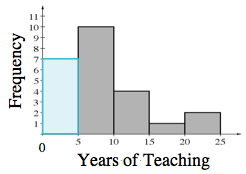### Home > CC3 > Chapter 9 > Lesson 9.2.7 > Problem9-153

9-153.
1.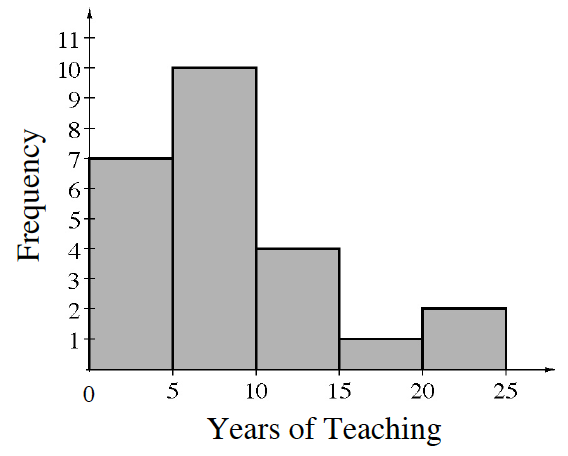A principal made the histogram at right to analyze how many years teachers had been teaching at her school. Homework Help ✎

1. How many teachers work at her school?

2. If the principal randomly chose one teacher to represent the school at a conference, what is the probability that the teacher would have been teaching at the school for 10 or more years? Write the probability in two different ways.

3. What is the probability that a teacher on the staff has been there for fewer than 5 years?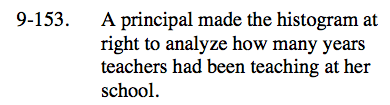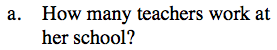Add up the frequencies represented by the bars. How many total teachers are there?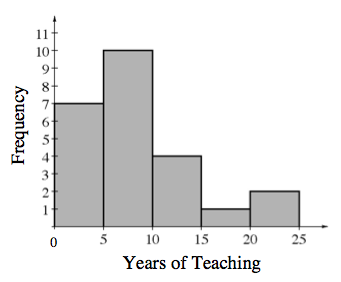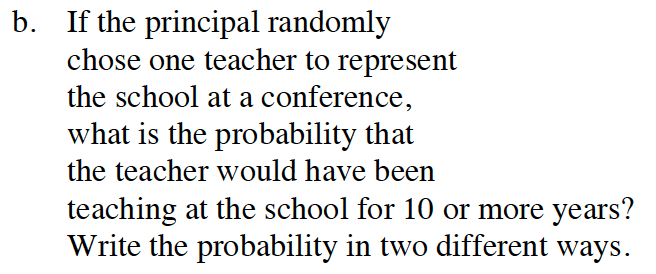Based on the histogram, how many teachers have been teaching for 10 years or more?

How can this probability be represented as a fraction and a percent?

$\frac{7}{24}\text{ or}\approx29 \%$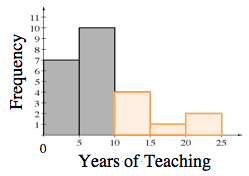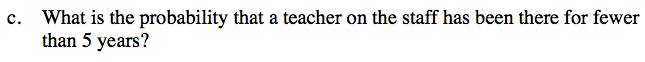Based on the histogram, how many teachers have been teaching for less than 5 years?